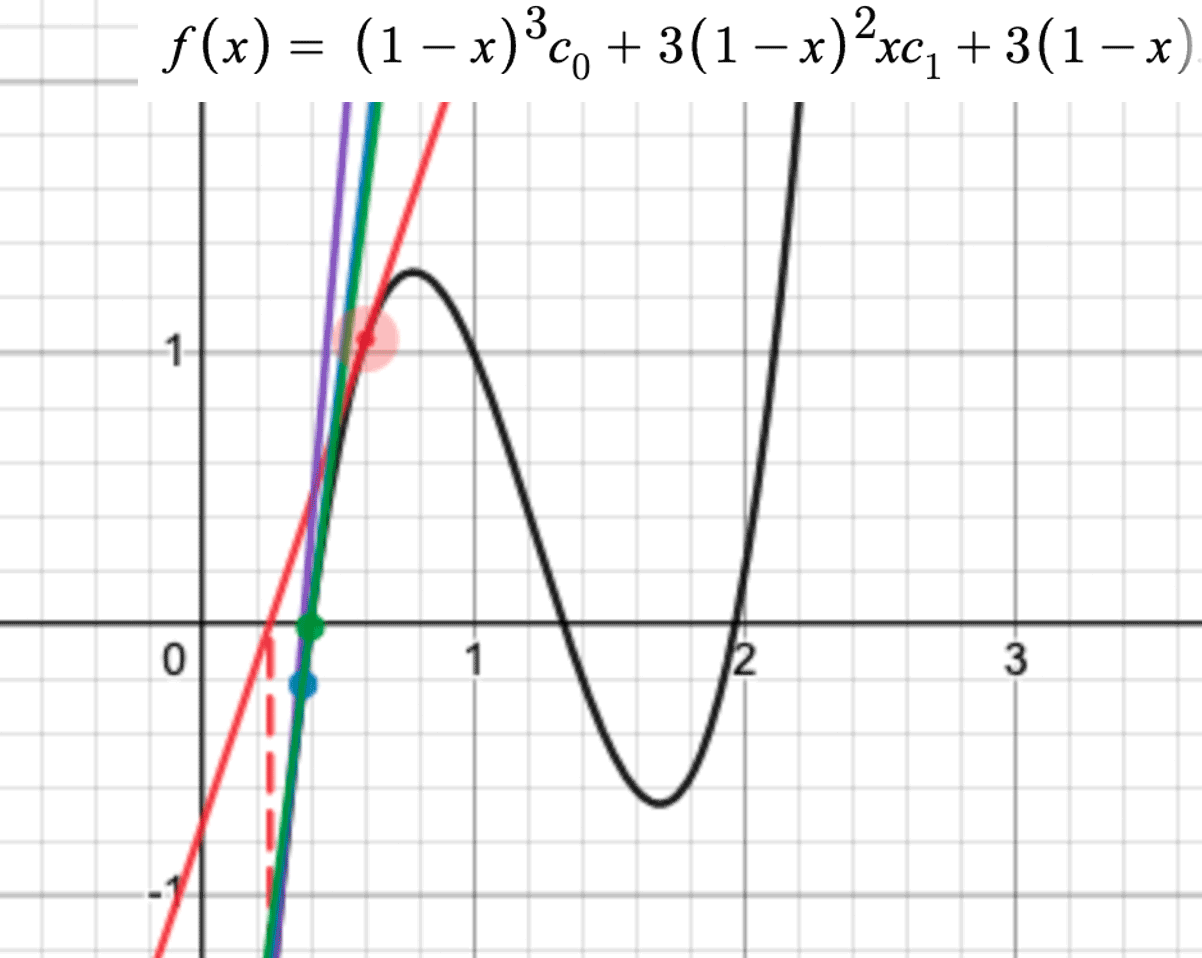# 理解贝塞尔曲线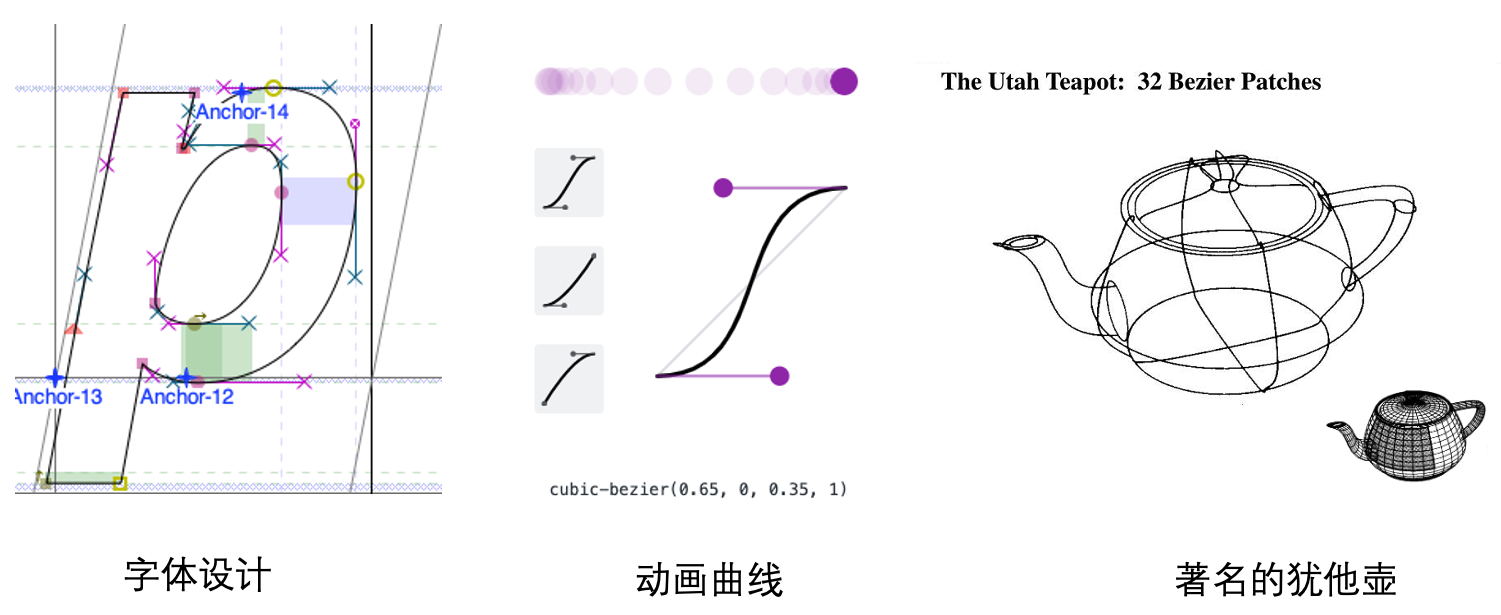## 起源介绍

### 为什么是三次曲线？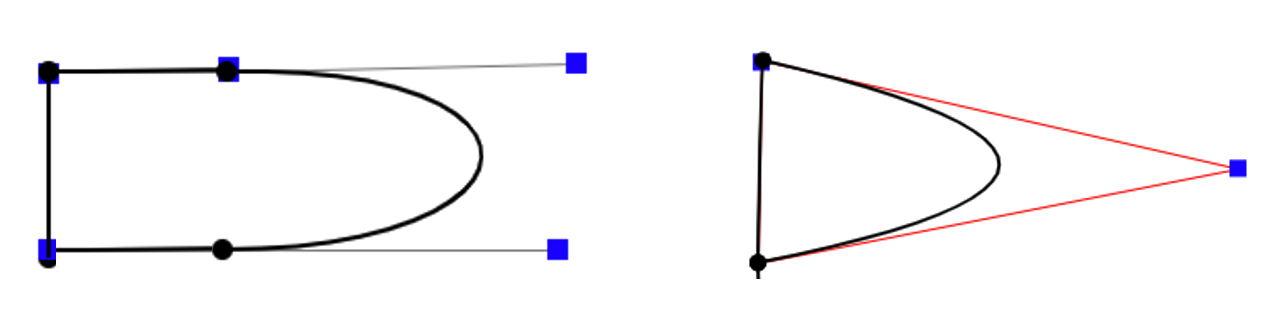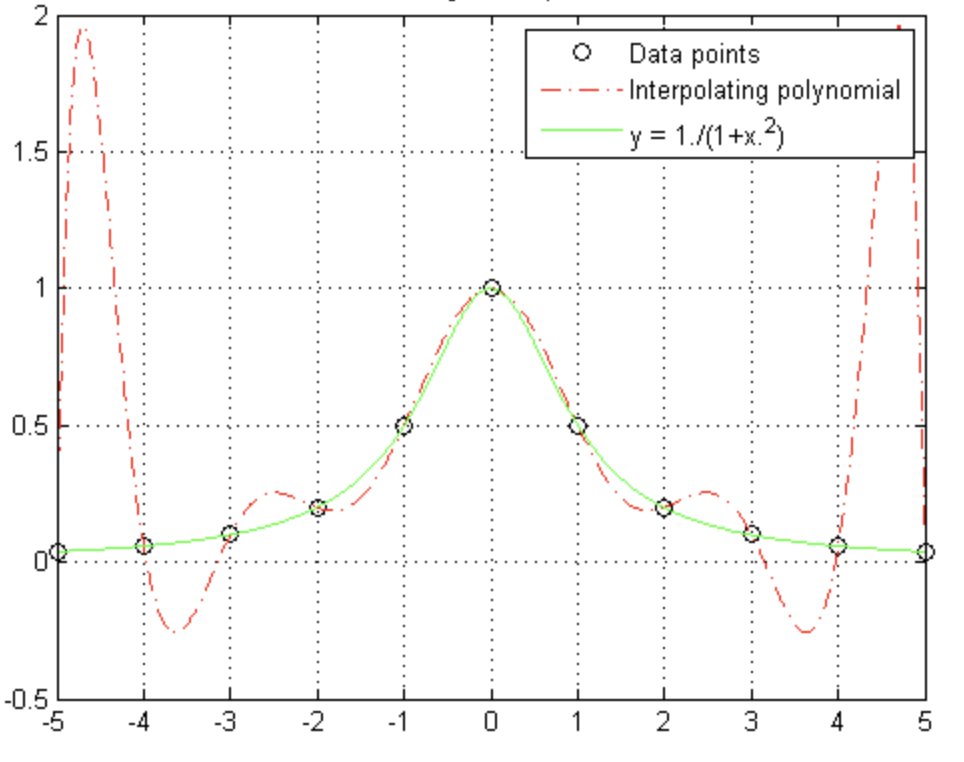### 为什么是贝塞尔？

y = a*x^3 + b*x^2 + c*x + d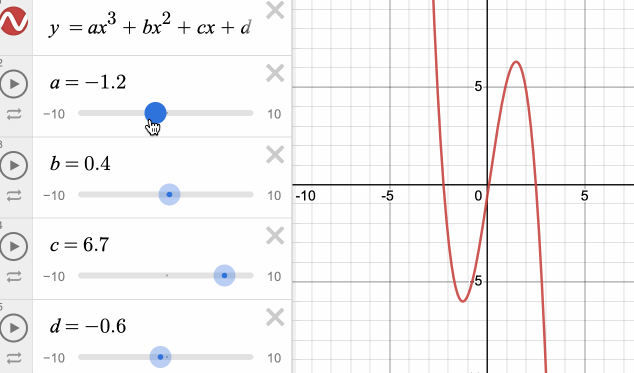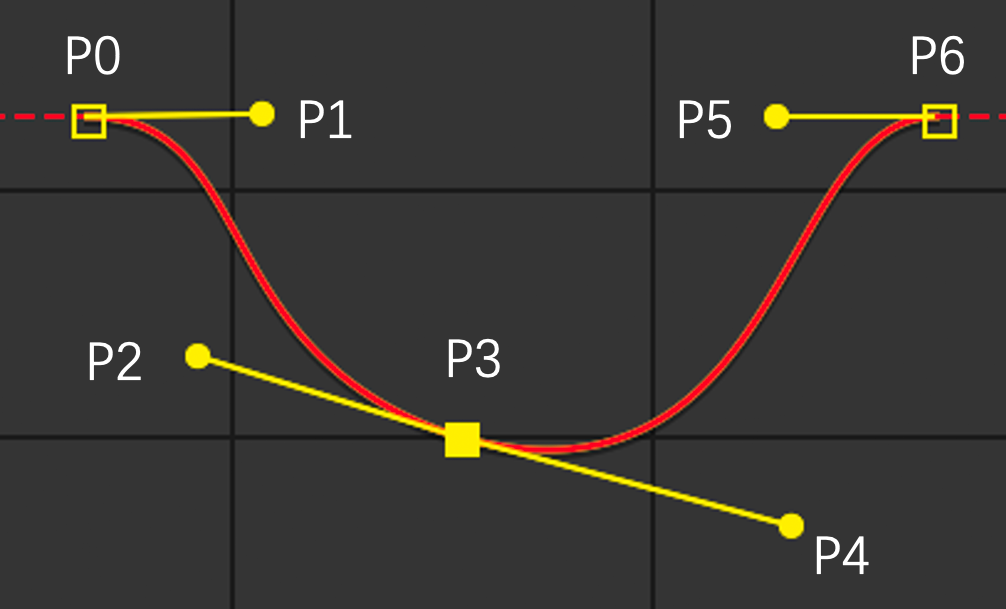CSS的cubic-bezier同样也是有四个控制点：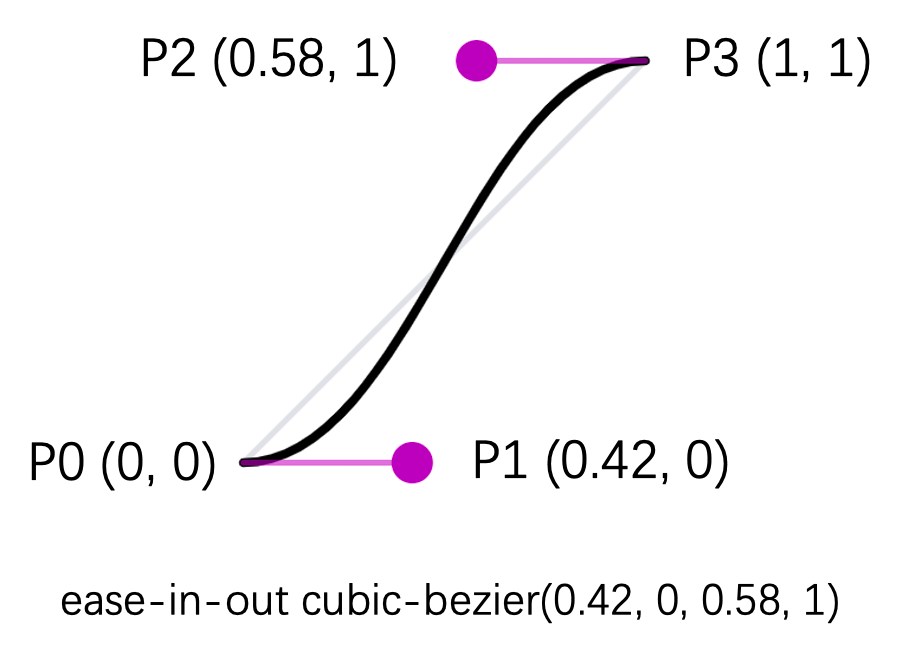CSS贝塞尔曲线的y坐标是一个相对单位，范围为[0, 1]。实际设定的属性值是在具体的CSS属性如transform中设定的，使用这个相对值结合实际的起始与终始值，就可以换算成真实的值，举例如下：
translateX = translateXBegin + (translateXEnd – translateXBegin) * y + translateXBegin

## 参数化曲线

x(t) = f(t)
y(t) = g(t)

x(t) = 1 – t
y(t) = 2t^2 + 1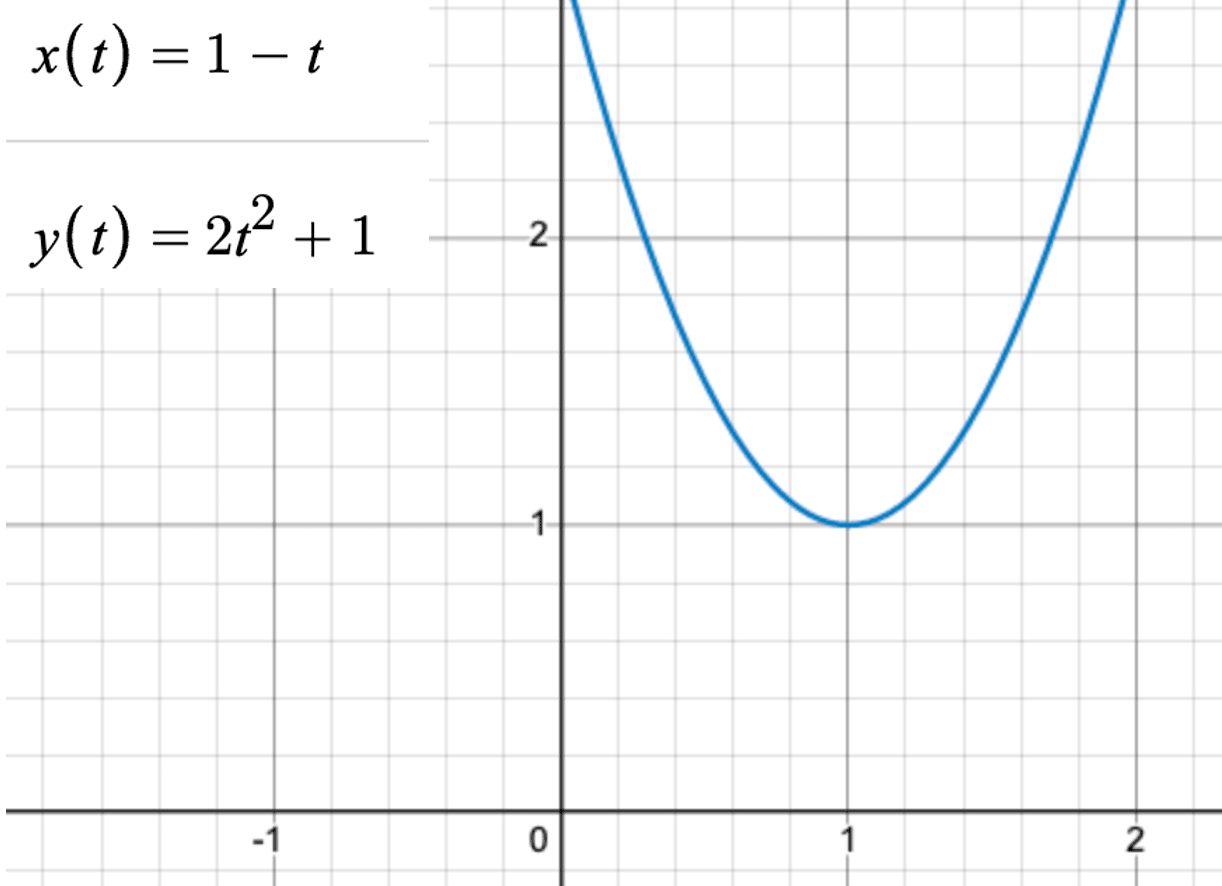y = 2x^2 + 4x – 1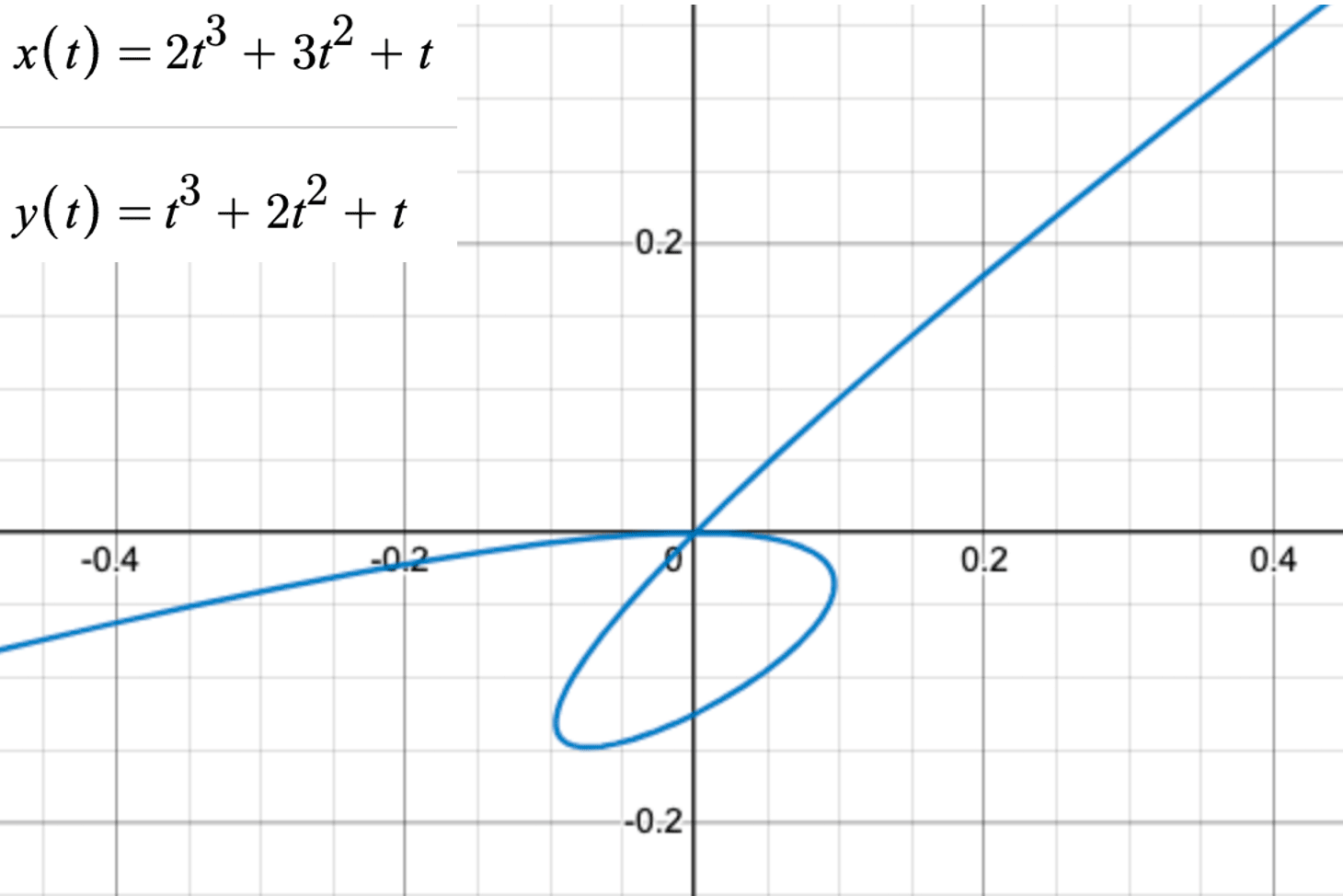x(t)是一个三次方程，三次方程有三个根，且可能会存在复根。将x(t)的根代入y(t)方程上，需要区分若干种情况。情况就会变得复杂。由此，参数化方程的一个重要意义是可以表示一般方程难以甚至无法表示的形式。

## N阶贝塞尔曲线

### 一阶贝塞尔曲线

x(t) = c0 + t(c1 – c0)
y(t) = v0 + t(v1 – v0)

B(t) = P0 + t(P1 – P0) = tP1 + (1 – t)P0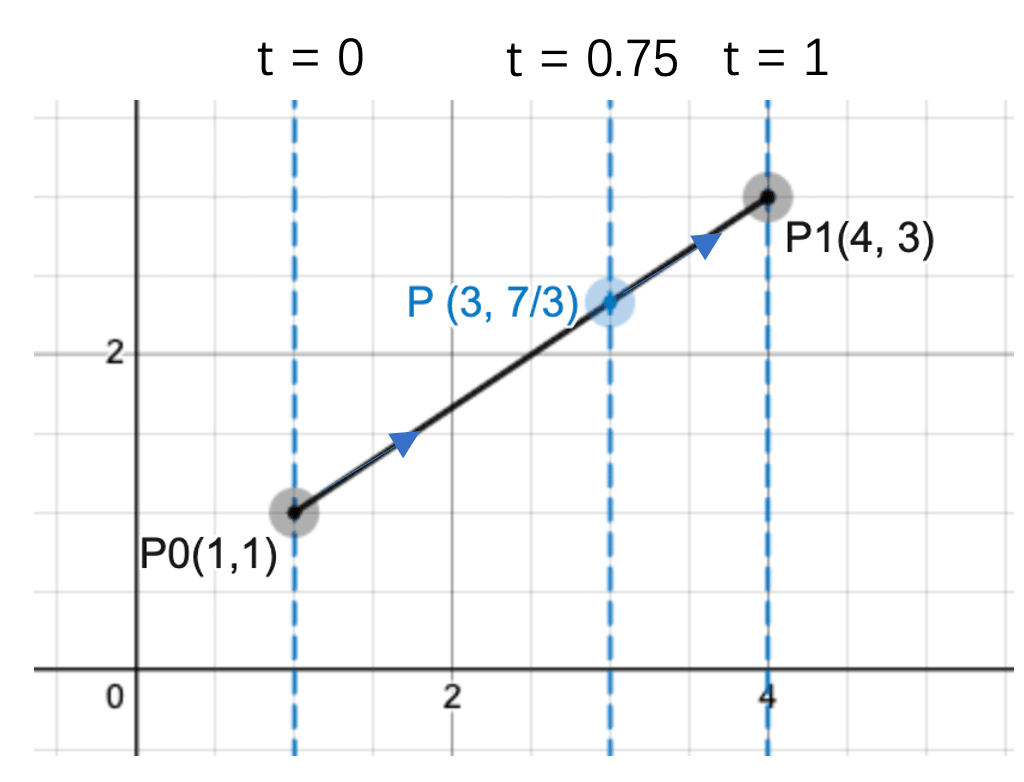（1）当t = 0时，求解可知曲线上的点为(1, 1);。
（2）当t = 0.25时，曲线上的点为（3, 7/3）。
（3）当t = 1时，曲线上的点为（4, 3）。

### 二阶贝塞尔曲线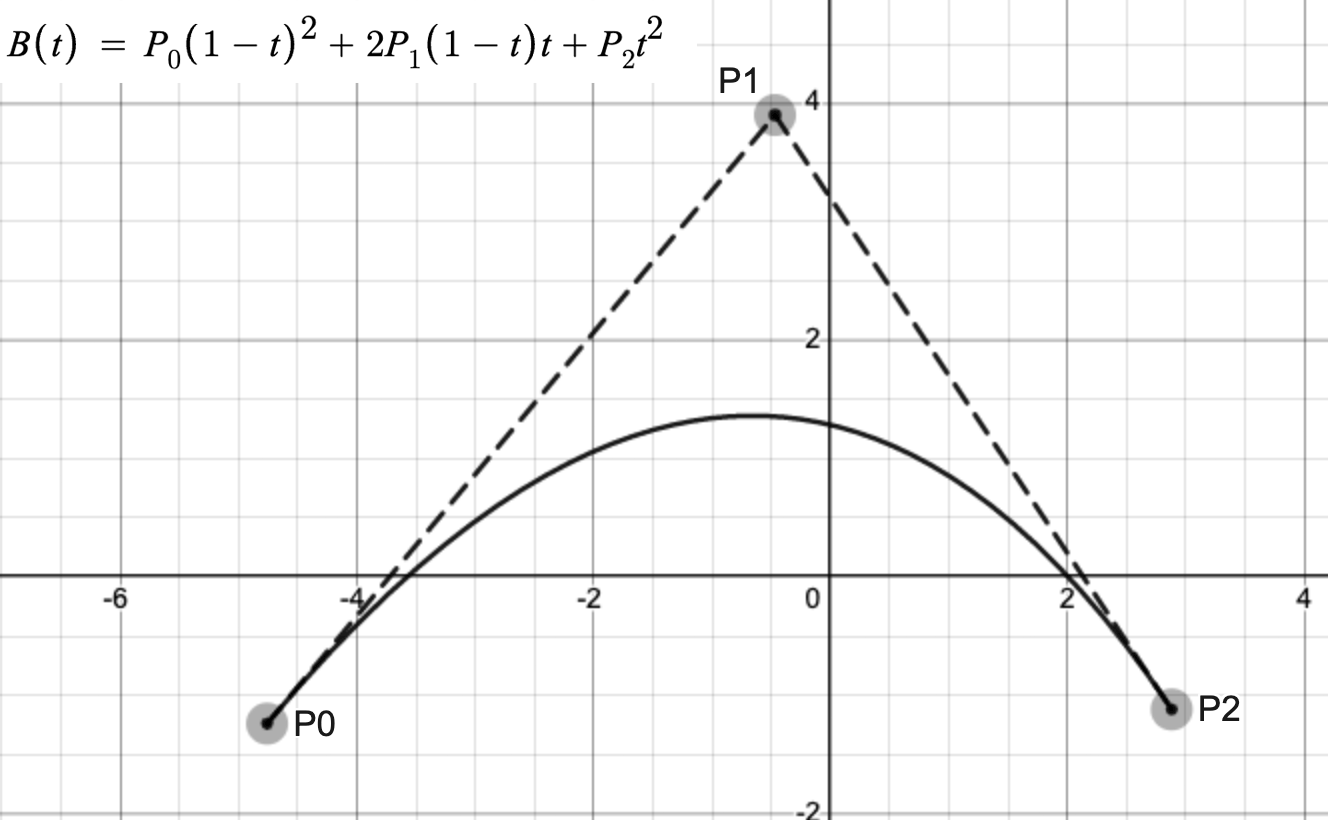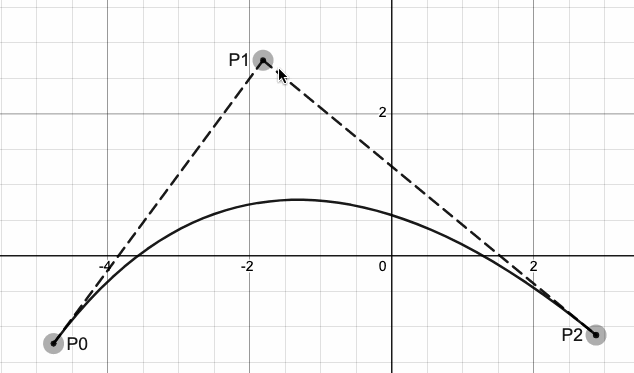（1）将线段LP0P1当作一阶贝塞尔曲线，根据t求解该曲线上的点为P3。

（2）将线段LP1P2当作一阶贝塞尔曲线，根据t求解该曲线上的点为P5。

（3）将线段LP3P4当作一阶贝塞尔曲线，根据t求解该曲线上的点为P4。P4即为二阶贝塞尔曲线上的点。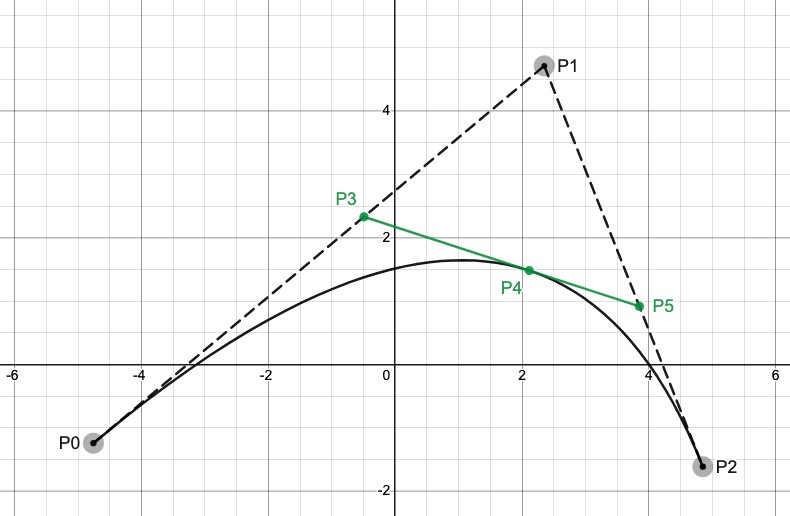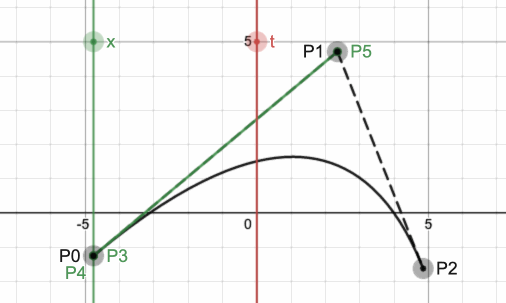lerp(P0, P1, t) = tP1 + (1 – t)P0

P3 = lerp(P0, P1, t) =  tP1 + (1 – t)P0
P5 = lerp(P1, P2, t) = tP2+ (1 – t)P1

P4 = lerp(P3, P5, t) = （1 – t)^2 P0 + 2t(1 – t)P1 + t^2 P2

### 三阶贝塞尔曲线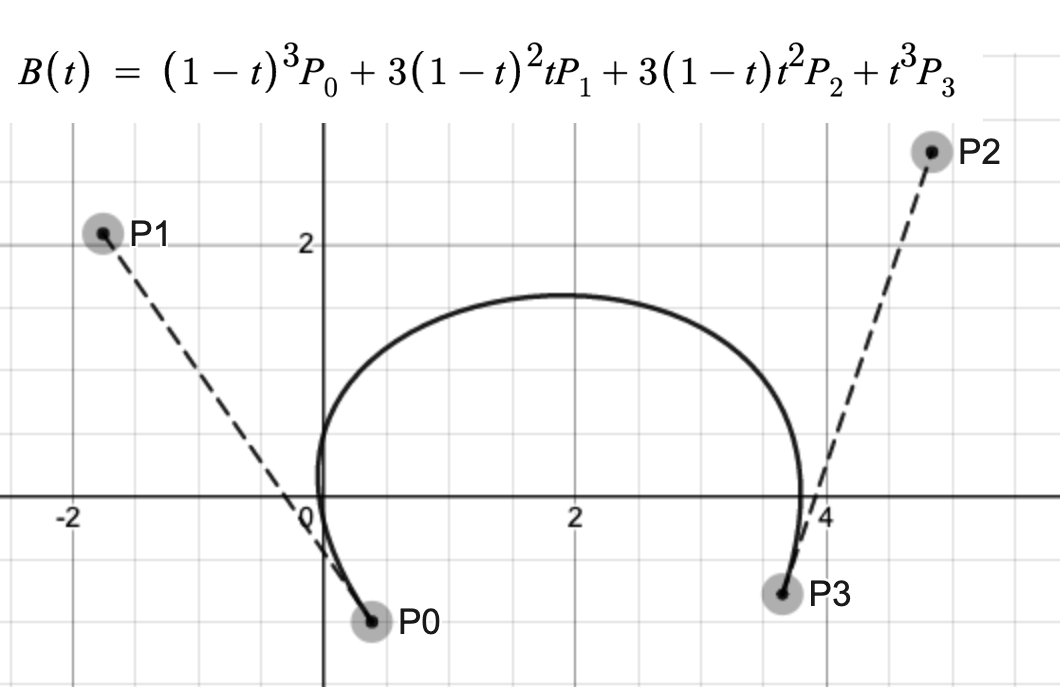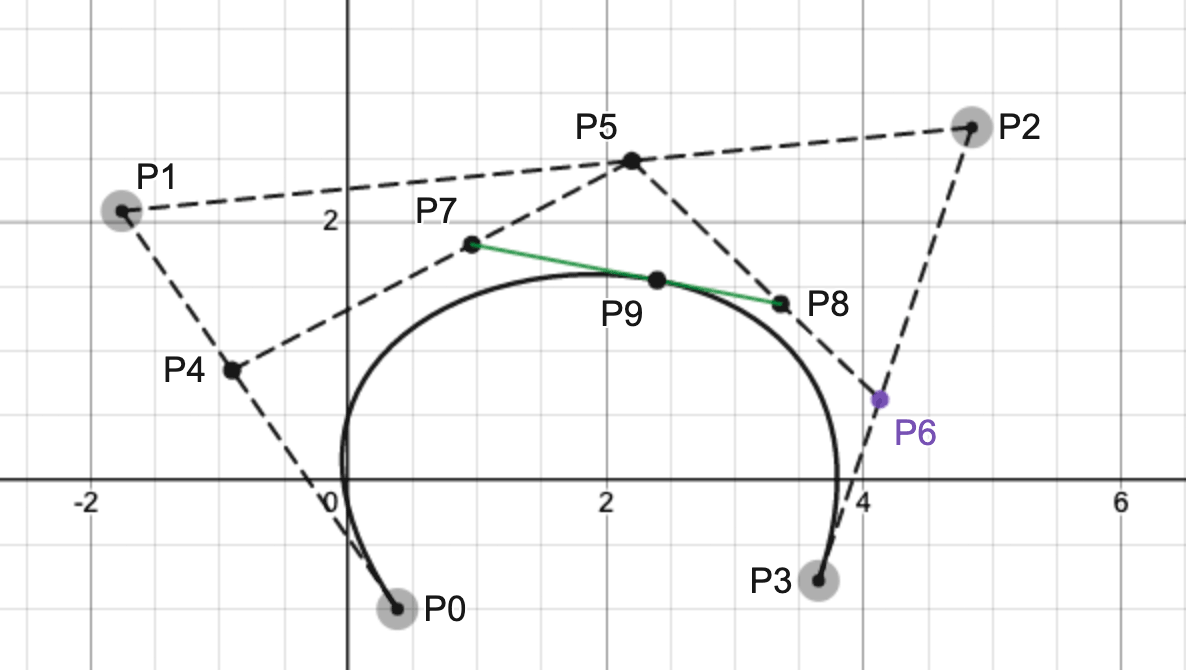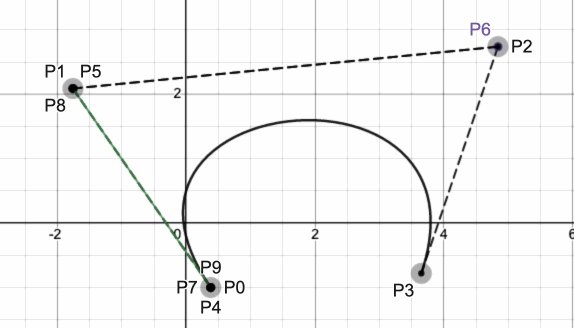## 动画贝塞尔曲线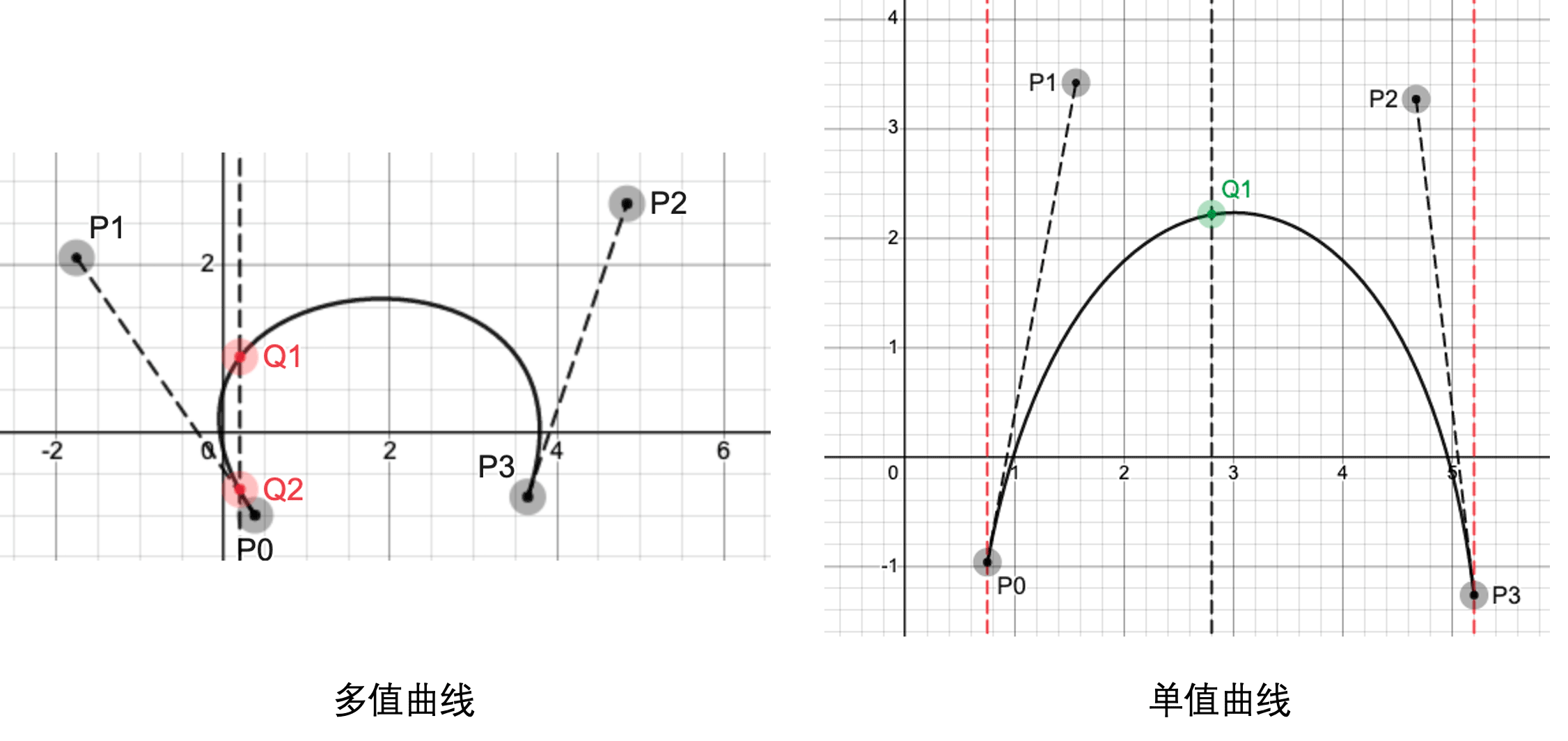## 动画贝塞尔曲线值求解

### 使用简单的曲线拟合

ease-in-out拟合的效果与真实的曲线对比如下：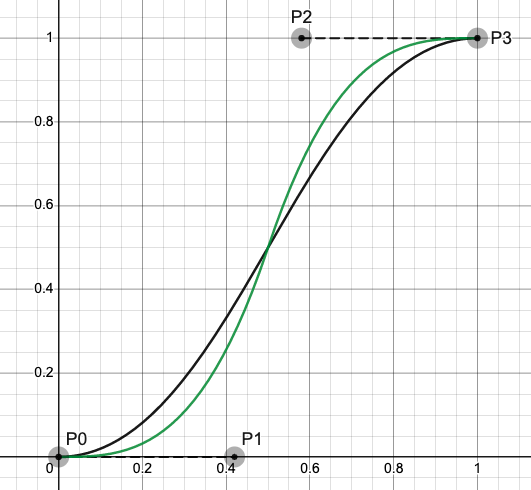### 牛顿迭代法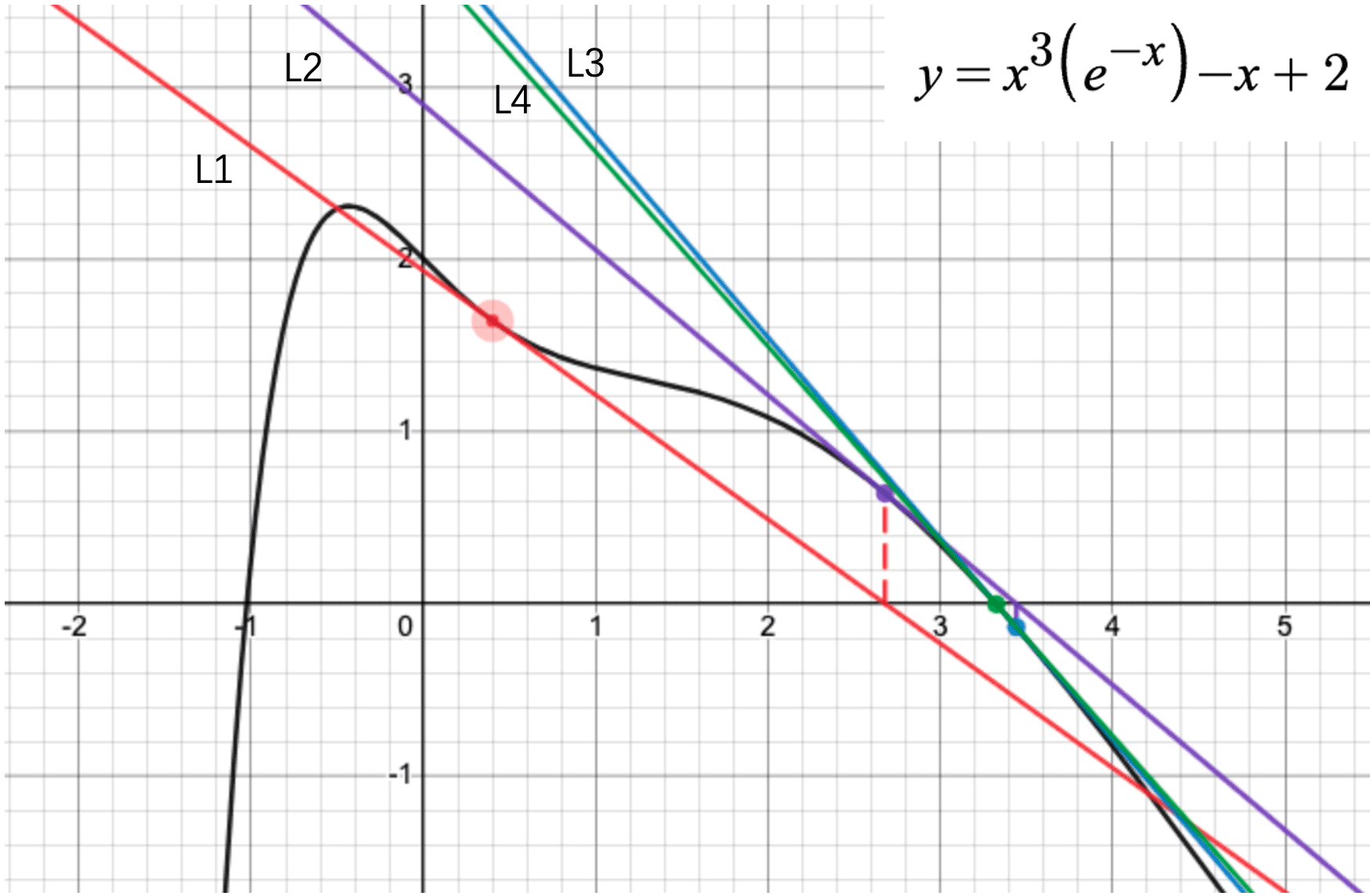（1）任意选取一个起始点，如图中的x1 = 0.4，计算y1 = f(x1)的值，该值与0相差较大，因此x = 0.4不是方程的解。

（2）作曲线在(x1, y1)上的切线L1，与x轴相交于点（x2, 0）。

（3）计算y2 = f(x2)，同样该值与0相差较大，也不是方程的解。

（4）作曲线在(x2, y2)上的切线L2，与x轴相交于点(x3, 0)。

（5）按同样的步骤得到切线L3与L4，由图可见f(x4)的值已经非常接近0（绿色的线与曲线的交点）.